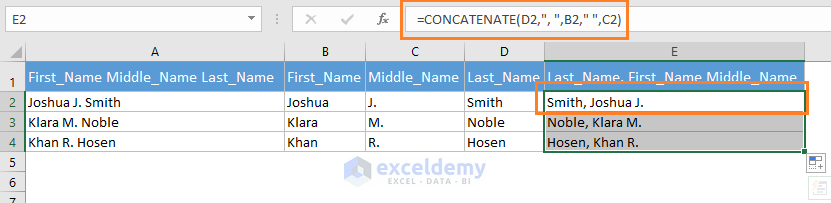Disclosure: This post may contain affiliate links, meaning when you click the links and make a purchase, we receive a commission.

# How to switch first and last name in Excel with comma (5 Easy Ways)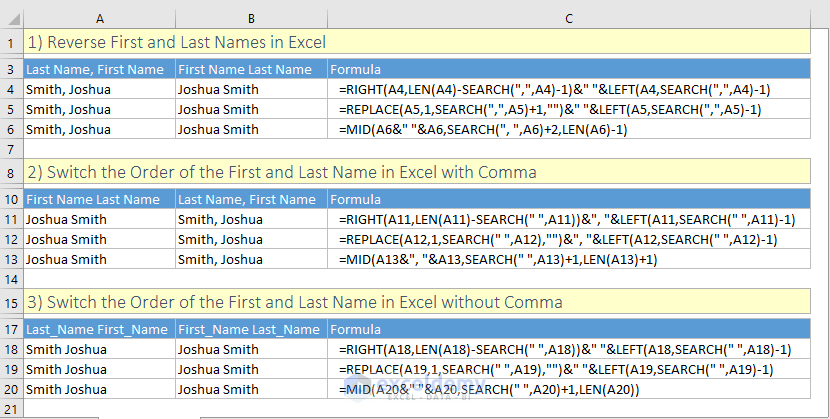In this article, I will show how to switch first and last names in Excel with a comma.

Depending on the problems, you might face many problems. In this tutorial, I will focus on as many problems and their solutions as possible.

But if you have a special problem and you’re facing problem to solve it, then let us know in the comment box. We shall try to give you a solution.

Switch-first-and-last-name-in-Excel-with-comma.xlsx

## 1) Reverse First and Last Names in Excel

In this method, we shall reverse First Name and Last Name in the following way:

Last Name, First Name = First Name Last Name

For example,

Smith, Joshua = Joshua Smith

We can do this using any of the following 3 Excel formulas:

`=RIGHT(A2,LEN(A2)-SEARCH(",",A2)-1)&" "&LEFT(A2,SEARCH(",",A2)-1)`

`=REPLACE(A3,1,SEARCH(",",A3)+1,"")&" "&LEFT(A3,SEARCH(",",A3)-1)`

`=MID(A4&" "&A4,SEARCH(", ",A4)+2,LEN(A4)-1)`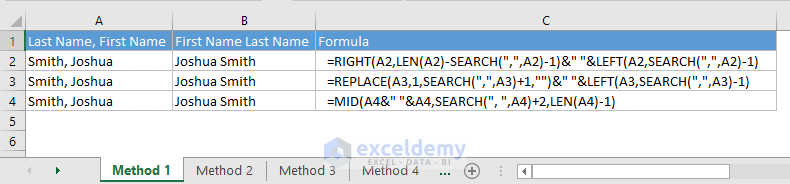## 2) Switch the Order of the First and Last Name in Excel with Comma

In this method, we shall switch the order of the first and last name in Excel with a comma like the following:

First Name Last Name = Last Name, First Name

For example,

Joshua Smith = Smith, Joshua

We can do this using any of the following Excel formulas:

`=RIGHT(A2,LEN(A2)-SEARCH(" ",A2))&", "&LEFT(A2,SEARCH(" ",A2)-1)`

`=REPLACE(A3,1,SEARCH(" ",A3),"")&", "&LEFT(A3,SEARCH(" ",A3)-1)`

`=MID(A4&", "&A4,SEARCH(" ",A4)+1,LEN(A4)+1)`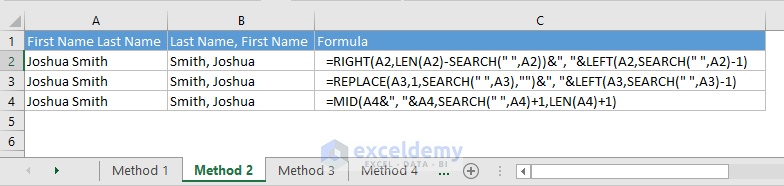## 3) Switch the Order of the First and Last Name in Excel without Comma

This is what we shall do in this method:

Last_Name First_Name = First_Name Last_Name

For example,

Smith Joshua = Joshua Smith

Any of the following formulas will do so:

`=RIGHT(A2,LEN(A2)-SEARCH(" ",A2))&" "&LEFT(A2,SEARCH(" ",A2)-1)`

`=REPLACE(A3,1,SEARCH(" ",A3),"")&" "&LEFT(A3,SEARCH(" ",A3)-1)`

`=MID(A4&" "&A4,SEARCH(" ",A4)+1,LEN(A4))`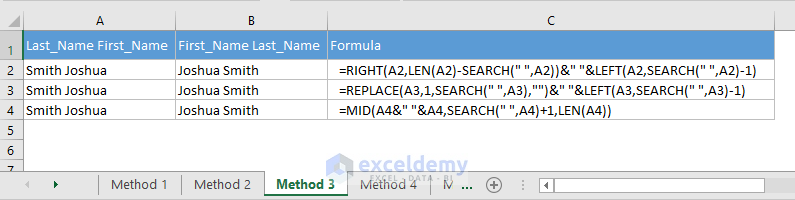## 4) Using Excel Flash Fill Feature (for Excel version 2013 and later)

Sometimes a name might have more than two parts. In this case, you can use Excel’s Flash Fill feature.

Keyboard Shortcut: CTRL + E

• At first, make your desired output in the cell B2. If necessary, make more outputs in some more cells in the column to give Excel a hint what your actual outputs will be.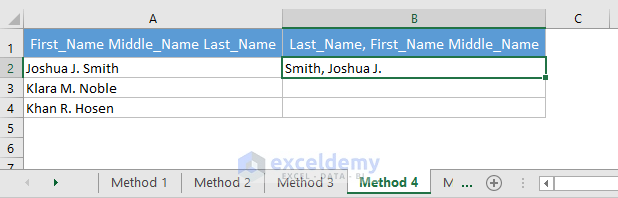• Now select that (B2) or those cells and press CTRL + E on your keyboard. You will find a drop-down named Flash Fill Options. Click on the drop-down and choose the Accept suggestions command if everything is OK. Or choose the Undo Flash Fill command if you want to cancel the outputs.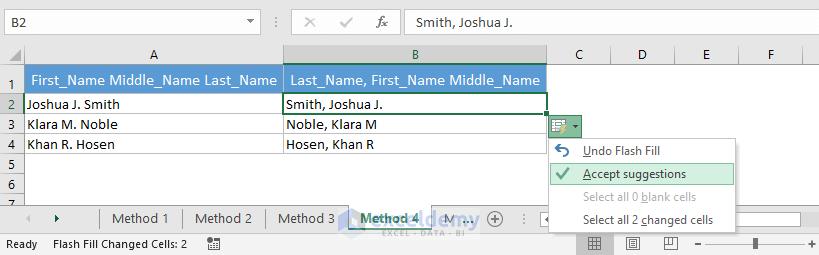## 5) Using Text to Columns Feature of Excel

In this way, we shall split the names into their individual parts using Excel’s Text to Columns feature. Then we shall concatenate those parts to make a new name format.

• Select the cells where the names are and then open the Text to Columns wizard of Excel (Data > Data Tools > Text to Columns)

Keyboard shortcut to open the Text to Columns wizard: ALT + A + E

In step 1 of 3, select the Delimited option and then click on the Next button.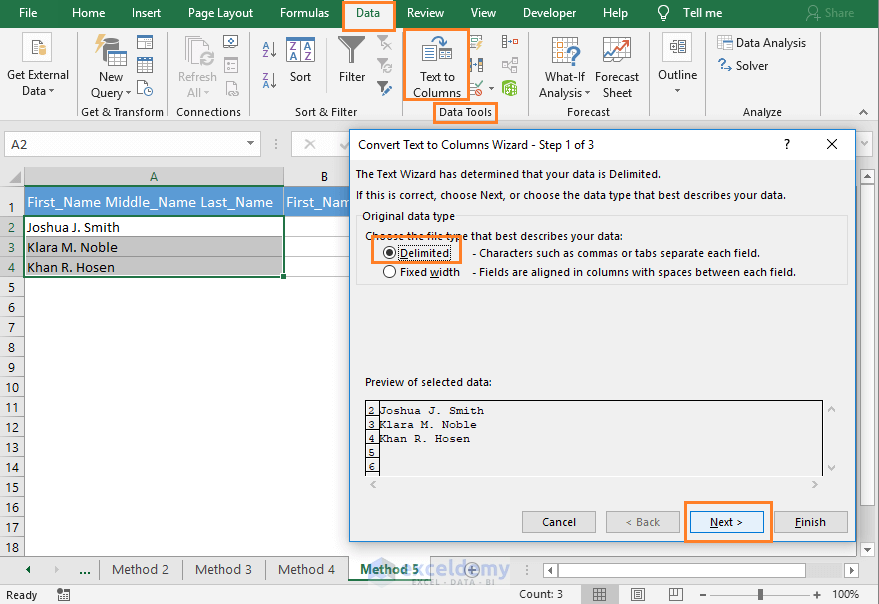• In step 2 of 3, select Space as the Delimiter. And click on the Next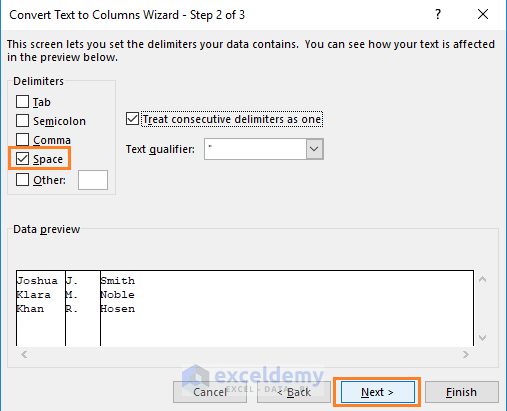• In step 3 of 3, we select the Destination cell as B2 and click on the Finish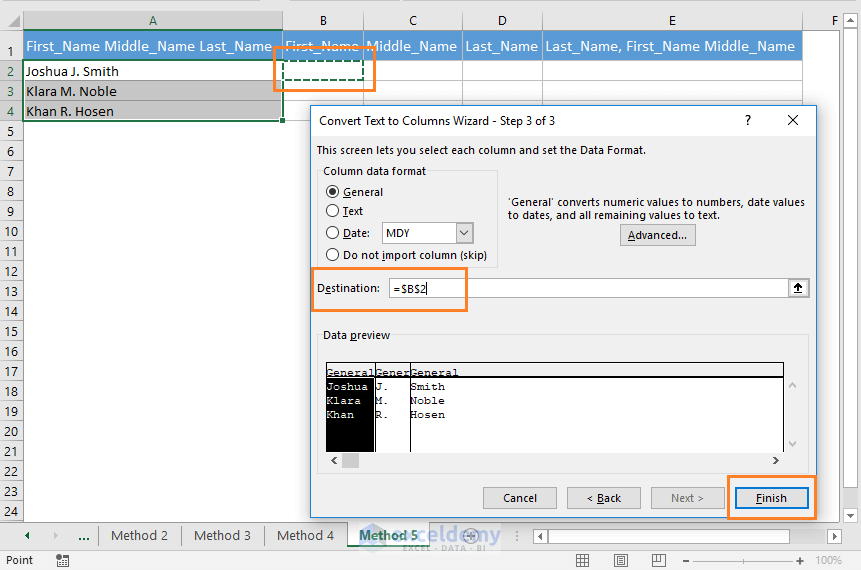• This is what we get at this stage: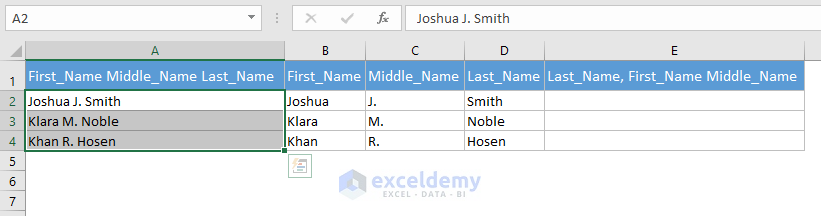• Now I have used the CONCATENATE function to join these cells and create a new name format.

`=CONCATENATE(D2,", ",B2," ",C2)`

But you can also use the & operator in this way:

`=D2&", "&B2&" "&C2`